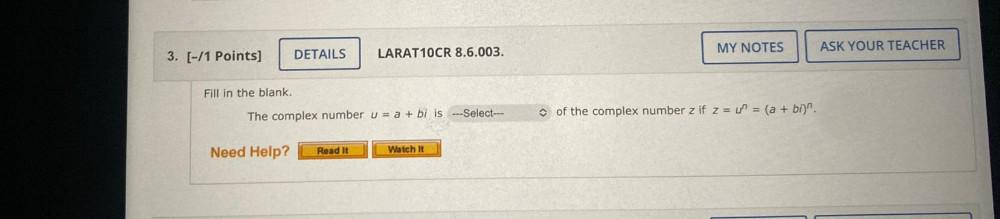Question:

# The complex number u = a + bi is_____________ of the complex number z if z = u^n = (a + bi)^n.The complex number u = a + bi is_____________ of the complex number z if z = u^n = (a + bi)^n.